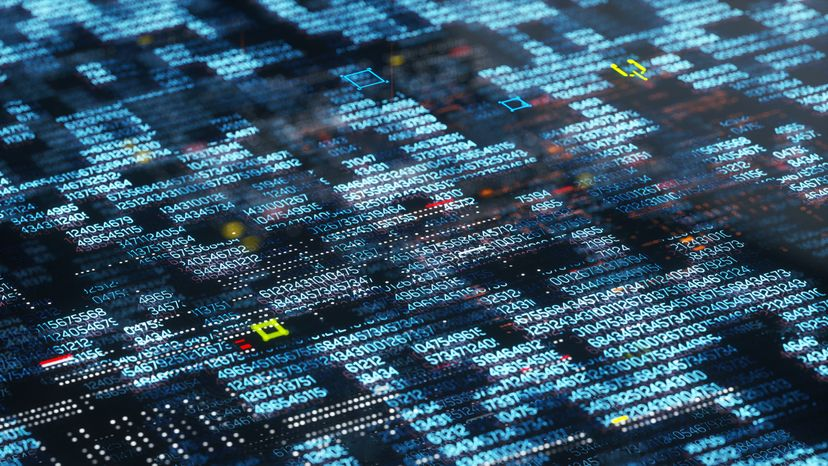# How can a totally logical machine like a computer generate a random number?Computer programming is a diverse topic. Andriy Onufriyenko / Getty Images

There are two ways that computers can generate random numbers:

You can create some sort of device that monitors a completely random natural event and sends its results to the computer. For example, you could place a piece of radioactive material in front of a Geiger counter and connect the Geiger counter to a computer. Since radioactive decay is random, the Geiger counter would create truly random numbers. This approach is pretty rare, because not many people have Geiger counters connected to their machines. You can create a formula that generates a pseudo-random number. When designing the formula, the idea is for it to produce a string of numbers that would look random to anyone who did not know what the formula is. Characteristics of a good formula include:

• No repetition: The sequence does not cycle around and repeat itself. Good numeric distribution: If the formula is producing random numbers between 0 and 9, the number of zeros, ones, twos, etc. that it produces should be roughly equal over a long period of time. Lack of predictability: You have no way to predict what the next number will be unless you know the formula and the seed (the initial value).

Here's an example of a simple random-number formula from the book "The C Programming Language," by Kernighan and Ritchie:

```int rand() {  random_seed = random_seed * 1103515245 +12345;
return (unsigned int)(random_seed / 65536) % 32768; }```

This formula assumes the existence of a variable called random_seed, which is initially set to some number. The random_seed variable is multiplied by 1,103,515,245 and then 12,345 gets added to the product; random_seed is then replaced by this new value. This is actually a pretty good pseudo-random number generator. It has a good distribution and it is non-repeating. If you use it to produce random numbers between 0 and 9, here are the first 20 values that it produces if the seed is 10:

`44607423505664567674`

If you have it produce 10,000 values between 0 and 9, here's the distribution:

`0 - 10151 - 10242 - 10483 - 9964 - 9885 - 10016 - 9967 - 10068 - 9659 - 961`

Any pseudo-random number formula depends on the seed value to start the sequence. If you start with the same seed, you will get the same sequence of values from the formula. So if you give the rand() function shown above the seed of 10 on one computer and look at the stream of numbers it produces, it will be identical to the stream of numbers produced on any computer that runs it with a seed of 10. In the case of the Global Positioning System, this reproducibility is used as a way to give each satellite a predictable but different pattern of values that the GPS receiver can track.

To create a random and unpredictable sequence, the seed must be a truly random number. To get this truly random number for the seed, most programs use the current date and time, converted to an integer value (for example, converted to the number of seconds that have elapsed since January 1, 1970). Since this is a different number every time you start the program, it makes a good seed.